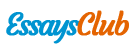# Investment Chapter - Importance of an Investment Policy Statement

Autor:   •  October 31, 2017  •  980 Words (4 Pages)  •  318 Views

Page 1 of 4

...

ROI is (6960-8000) / 24000 = -4.33%

Price decreases to \$32

Assets

Liabilities and Account Equity

500 shares

\$16000

Margin loan

\$17040

Account equity

\$-1040

Total

\$16000

Total

\$16000

ROI is (16000-24000) / 24000 = -33%

If simply purchased \$8000 without margin, then spend \$7968 to buy 166 shares with \$48 per share.

If the price increases to \$53 one year after, the ROI will be 53*166 – 7968 / 7968 = 10.42%

If the price remains \$48, then ROI is 0 since there is no margin.

If the price decreases to \$32, ROI should be 32*166-7968 / 7968 = -33.33%

#22

After selling the stock and gaining dividend, margin loan interest rate is 6%+1.25%=7.25%. Since you hold the stock for six months, so after six months, the margin maintenance should be 16600* (1+7.25%/2) = 17201.75. The selling price is \$53, with a dividend of \$0.25 per share, thus the value of 600 shares after six months should be \$53.25*600=31950

Assets

Liabilities and Account Equity

600 shares

\$31950

Margin loan

\$17201.75

Account equity

\$14748.25

Total

\$31950

Total

\$31950

Total dollar return = 0.25*600 + (14748.25-11000) = 150+3748.25=\$3898.25

#25

One year before

Assets

Liabilities and Account Equity

750 shares

\$72000

Margin loan

\$43200

Account equity

\$28800

Total

\$72000

Total

\$72000

One year late, covering short when the price hits \$86.5

Assets

Liabilities and Account Equity

750 shares

\$64875

Margin loan

\$43200

Account equity

\$21675

Total

\$64875

Total

\$64875

Short: (72000-64875) – (0.75*750*2) = 6000

ROI= (72000-64875-1125-72000)/72000 = -91.67%

#26

Assets

Liabilities and Account Equity

600 shares

\$43200

Margin loan

\$21600

Account equity

\$21600

Total

\$43200

Total

\$43200

Price of \$63

Assets

Liabilities and Account Equity

600 shares

\$37800

Margin loan

\$21600

Account equity

\$16200

Total

\$37800

Total

\$37800

Margin = 21600/ 37800 = 57.14%

Price of \$77

Assets

Liabilities and Account Equity

600 shares

\$46200

Margin loan

\$21600

Account equity

\$24600

Total

\$46200

Total

\$46200

Margin = 21600/ 46200 = 46.75%

Then calculate EAR at the price of \$63

N=5, PV=- 43200, FV=37800 , PMT=0, thus I/Y= -2.63%

APR= -2.63% *12 = -31.62% thus EAR=(1-2.63%)12 -1= -27.37%

EAR at the price of \$77

N=5,

...